# Diversify ESG Portfolios

This example shows how to include qualitative factors for environmental, social, and corporate governance (ESG) in the portfolio selection process. The example extends the traditional mean-variance portfolio using a `Portfolio` object to include the ESG metric. First, the `estimateFrontier` function computes the mean-variance efficient frontier for different ESG levels. Then, the example illustrates how to combine the ESG performance measure with portfolio diversification techniques. Specifically, it introduces hybrid models that use the Herfindahl-Hirshman (HH) index and the most diversified portfolio (MDP) approach using the `estimateCustomObjectivePortfolio` function. Finally, the `backtestEngine` framework compares the returns and behavior of the different ESG strategies.

### Define Mean-Variance Portfolio

Load the table with the asset returns and a vector with ESG scores for the assets. Both the asset returns and their ESG scores are simulated values and do not represent the performance of any real securities. However, you can apply the code and workflow in this example to any data set with prices and returns and ESG information.

```% Load data load('asset_return_100_simulated.mat') % Returns table load('ESG_s26.mat') % ESG scores```

Transform the returns table into a prices `timetable`.

```% Transform returns to prices assetPrices = ret2tick(stockReturns); % Transform prices table to timetable nRows = size(stockReturns,1); day = datetime("today"); Time = (day-nRows):day; assetPrices = table2timetable(assetPrices,"RowTimes",Time);```

Define a `Portfolio` object with default constraints where the weights must be nonnegative and sum to 1.

```% Create a mean-variance Portfolio object with default constraints p = Portfolio; p = estimateAssetMoments(p,stockReturns); p = setDefaultConstraints(p);```

### Compute the Mean-Variance Efficient Frontier for Different ESG Levels

Obtain contour plots of the ESG-mean-variance efficient surface. You obtain the efficient surface from the Pareto optima of the multiobjective problem that includes all the performance metrics: average ESG score, average return, and return variance.

First, obtain feasible values of the ESG metric by finding the minimum and maximum ESG levels. To do this step, use the `estimateCustomObjectivePortfolio` function assigning the average ESG score as the objective function. The average ESG score of a portfolio is the weighted sum of the individual asset ESG scores, where the weights are given by the amount invested in each asset.

```% Define objective function: average ESG score ESGscore = @(x) ESGnumeric'*x; % Find the minimum ESG score solMin = estimateCustomObjectivePortfolio(p,ESGscore); minESG = ESGscore(solMin)```
```minESG = 0.0187 ```
```% Find the maximum ESG score solMax = estimateCustomObjectivePortfolio(p,ESGscore, ... ObjectiveSense="maximize"); maxESG = ESGscore(solMax)```
```maxESG = 0.9735 ```

To compute the contours of the efficient surface, you add the average ESG score as a constraint using the `setInequality` function. The coefficients of the linear constraint are the ESG scores associated with each asset and the right-hand side is the target ESG score for the desired contour. Notice that the convention of the inequalities in the `Portfolio` object is $\le$. Because the goal is to maximize the average ESG score, the target ESG value for the contour should be a lower bound. Therefore, you need to flip the signs of the coefficients and the right-hand side of the added inequality. The function that computes and plots the contours is in the Local Functions section.

```N = 20; % Number of efficient portfolios per ESG value % Add ESG score as a constraint to the Portfolio object Ain = -ESGnumeric'; bin = -minESG; % Start with the smallest ESG score p = setInequality(p,Ain,bin); % Esimate lower value for contour plots pwgt = estimateFrontier(p,N); pESG = pwgt'*ESGnumeric; minContour = max(pESG); % All ESG scores lower than this have % overlapped contours. % Plot contours nC = 5; % Number of contour plots plotESGContours(p,ESGnumeric,minContour,maxESG,nC,N);```### Diversification Techniques

In this example, the two diversification measures are the equally weighted (EW) portfolio and the most diversified portfolio (MDP).

You obtain the EW portfolio using the Herfindahl-Hirschman (HH) index as the diversification measure

`$\mathrm{HH}\left(\mathit{x}\right)=\sum _{\mathit{i}=1}^{\mathit{n}}{\mathit{x}}_{\mathit{i}}^{2}$`

An equally weighted portfolio minimizes this index. Therefore, the portfolios that you obtain from using this index as a penalty have weights that satisfy the portfolio constraints and are more evenly weighted.

The diversification measure for the most diversified portfolio (MDP) is

`$\mathrm{MDP}\left(\mathit{x}\right)=-\sum _{\mathit{i}=1}^{\mathit{n}}{\sigma }_{\mathit{i}}{\mathit{x}}_{\mathit{i}}$`

where ${\sigma }_{\mathit{i}}$ represents the standard deviation of asset $\mathit{i}$. Using this measure as a penalty function maximizes the diversification ratio .

`$\phi \left(\mathit{x}\right)=\text{\hspace{0.17em}}\frac{{\mathit{x}}^{\mathit{T}}\sigma }{\sqrt{{\mathit{x}}^{\mathit{T}}\Sigma \mathit{x}}}$`

If the portfolio is fully invested in one asset, or if all assets are perfectly correlated, the diversification ratio $\phi \left(\mathit{x}\right)$ is equal to 1. For all other cases, $\phi \left(\mathit{x}\right)>1$. Therefore, if $\phi \left(\mathit{x}\right)\approx 1$, there is no diversification, so the goal is to maximize $\phi \left(\mathit{x}\right)$. Unlike the HH index, the goal of MDP is not to obtain a portfolio whose weights are evenly distributed among all assets, but to obtain a portfolio whose selected (nonzero) assets have the same correlation to the portfolio as a whole.

### Diversification for Fixed ESG Level

You can extend the traditional minimum variance portfolio problem subject to an expected return to include the ESG metric by setting an ESG constraint for the problem. The purpose of the ESG constraint is to force the portfolio to achieve an ESG score greater than a certain target. Then, add a penalty term to the objective function to guide the problem toward more or less diversified portfolios. How diversified a portfolio is depends on your choice of the penalty parameter.

Choose an ESG level of `0.85` and an expected return of `0.001`.

```% Minimum ESG and return levels ESG0 = 0.85; ret0 = 1e-3;```

Currently, the portfolio problem assumes that the weights must be nonnegative and sum to 1. Add the requirement that the return of the portfolio is at least `ret0` and the ESG score is at least `ESG0`. The feasible set is represented as $\mathit{X}$, which is

`$\mathit{X}=\left\{\mathit{x}\text{\hspace{0.17em}}|\text{\hspace{0.17em}}\mathit{x}\ge 0,\text{\hspace{0.17em}}\sum _{\mathit{i}=1}^{\mathit{n}}{\mathit{x}}_{\mathit{i}}=1,\text{\hspace{0.17em}}{\mu }^{\mathit{T}}\mathit{x}\ge {\mathit{ret}}_{0},\text{\hspace{0.17em}}\mathrm{ESG}\left(\mathit{x}\right)\ge {\mathit{ESG}}_{0}\right\}$`

Add the ESG constraint using the `setInequality` function.

```% Add ESG constraint Ain = -ESGnumeric'; bin = -ESG0; % Set target ESG score p = setInequality(p,Ain,bin);```

To add the return constraint, pass the name-value argument `TargetReturn=ret0` to the `estimateCustomObjectivePortfolio` function for each of the different custom objective portfolios of interest.

The portfolio that minimizes the variance with the HH penalty is

`$\underset{\mathit{x}\in \mathit{X}}{\mathrm{min}}\text{\hspace{0.17em}\hspace{0.17em}\hspace{0.17em}}{\mathit{x}}^{\mathit{T}}\Sigma \mathit{x}+{\lambda }_{\mathrm{HH}}{\mathit{x}}^{\mathit{T}}\mathit{x}$`

```% HH penalty parameter lambdaHH =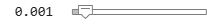0.001; % Variance + Herfindahl-Hirschman (HH) index var_HH = @(x) x'*p.AssetCovar*x + lambdaHH*(x'*x); % Solution that accounts for risk and HH diversification wHHMix = estimateCustomObjectivePortfolio(p,var_HH,TargetReturn=ret0);```

The portfolio that minimizes the variance with the MDP penalty is

`$\underset{\mathit{x}\in \mathit{X}}{\mathrm{min}}\text{\hspace{0.17em}\hspace{0.17em}\hspace{0.17em}}{\mathit{x}}^{\mathit{T}}\Sigma \mathit{x}-{\lambda }_{\mathrm{MDP}}\text{\hspace{0.17em}}{\sigma }^{\mathit{T}}\mathit{x}$`

```% MDP penalty parameter lambdaMDP =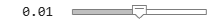0.01; % Variance + Most Diversified Portfolio (MDP) sigma = sqrt(diag(p.AssetCovar)); var_MDP = @(x) x'*p.AssetCovar*x - lambdaMDP*(sigma'*x); % Solution that accounts for risk and MDP diversification wMDPMix = estimateCustomObjectivePortfolio(p,var_MDP,TargetReturn=ret0);```

Plot the asset allocation from the penalized strategies.

```% Plot asset allocation figure t = tiledlayout(1,2); % HH penalized method nexttile bar(wHHMix'); title('Variance + HH') % MDP penalized method nexttile bar(wMDPMix') title('Variance + MDP') % General labels ylabel(t,'Asset Weight') xlabel(t,'Asset Number')```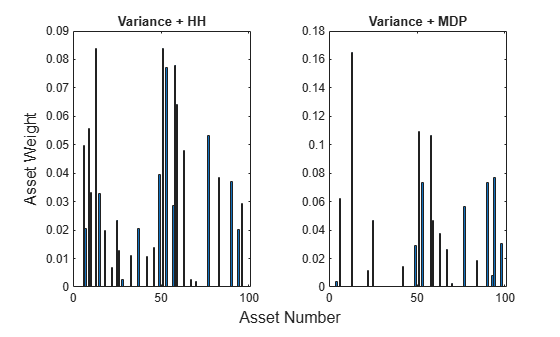The strategies that include the penalty function in the objective give weights that are between the minimum variance portfolio weights and the weights from the respective maximum diversification technique. In fact, for the problem with the HH penalty, choosing ${\lambda }_{\mathrm{HH}}=0$ returns the minimum variance solution, and as ${\lambda }_{\mathrm{HH}}\to \infty$, the solution approaches the one that maximizes HH diversification. For the problem with the MDP penalty, choosing ${\lambda }_{\mathrm{MDP}}=0$ also returns the minimum variance solution, and there exists a value ${\stackrel{ˆ}{\lambda }}_{\mathrm{MDP}}$ such that the MDP problem and the penalized version are equivalent. Consequently, values of ${\lambda }_{\mathrm{MDP}}\in \left[0,{\stackrel{ˆ}{\lambda }}_{\mathrm{MDP}}\right]$ return asset weights that range from the minimum variance behavior to the MDP behavior.

### Diversification by "Tilting"

The strategies in Diversification for Fixed ESG Level explicitly set a target ESG average score. However, a different set of strategies controls the ESG score in a less direct way. The method in these strategies uses ESG tilting. With tilting, you discretize the ESG score into `'high'` and `'low'` levels and the objective function penalizes each level differently. In other words, you use the diversification measure to tilt the portfolio toward higher or lower ESG values. Therefore, instead of explicitly requiring that the portfolios maintain a target ESG average score, you select assets, with respect to their ESG score, implicitly through the choice of penalty parameters.

Start by labeling the assets with an ESG score less than or equal to `0.5` as `'low'` and assets with an ESG score greater than `0.5` as `'high'`, and then remove the ESG constraint.

```% Label ESG data ESGlabel = discretize(ESGnumeric,[0 0.5 1], ... "categorical",{'low','high'}); % Create table with ESG scores and labels ESG = table(ESGnumeric,ESGlabel); % Remove ESG constraint p = setInequality(p,[],[]);```

The tilted version of the portfolio with the HH index is

`$\begin{array}{l}\mathrm{min}\text{\hspace{0.17em}\hspace{0.17em}\hspace{0.17em}}{\mathit{x}}^{\mathit{T}}\Sigma \mathit{x}+{\lambda }_{\mathrm{HH}}^{\mathrm{high}}\sum _{\mathit{i}\in \mathit{H}}{\mathit{x}}_{\mathit{i}}^{2}+{\lambda }_{\mathrm{HH}}^{\mathrm{low}}\sum _{\mathit{i}\in \mathit{L}}{\mathit{x}}_{\mathit{i}}^{2}\\ \mathrm{s}.\mathrm{t}.\text{\hspace{0.17em}\hspace{0.17em}}\text{\hspace{0.17em}}\sum _{\mathit{i}=1}^{\mathit{n}}{\mathit{x}}_{\mathit{i}}=1,\text{\hspace{0.17em}}{\mu }^{\mathit{T}}\mathit{x}\ge {\mathit{ret}}_{0},\text{\hspace{0.17em}}\mathit{x}\ge 0\text{\hspace{0.17em}}\end{array}$`

The feasible region does not bound the average ESG level of the portfolio. Instead, you implicitly control the ESG score by applying different penalization terms to assets with a `'high'` ESG score ($\mathit{i}\in \mathit{H}$) and assets with a `'low'` ESG score ($\mathit{i}\in \mathit{L}$). To achieve a portfolio with a high average ESG score, the penalty terms must satisfy that $0\le {\lambda }_{\mathrm{HH}}^{\mathrm{high}}\le {\lambda }_{\mathrm{HH}}^{\mathrm{low}}$.

```% HH penalty parameters % Penalty parameter for assets with 'low' ESG score lambdaLowHH =0.01; % Penalty parameter for assets with 'high' ESG score lambdaHighHH =0.001; % Lambda for HH 'tilted' penalty lambdaTiltHH = (ESG.ESGlabel=='low').*lambdaLowHH + (ESG.ESGlabel=='high').*lambdaHighHH; % Variance + Herfindahl-Hirschman (HH) index tilt_HH = @(x) x'*p.AssetCovar*x + lambdaTiltHH'*(x.^2); % Solution that minimizes variance + HH term wTiltHH = estimateCustomObjectivePortfolio(p,tilt_HH,TargetReturn=ret0);```

Similarly, the tilted version of the portfolio with the MDP penalty term is given by

`$\begin{array}{l}\mathrm{min}\text{\hspace{0.17em}\hspace{0.17em}\hspace{0.17em}}{\mathit{x}}^{\mathit{T}}\Sigma \mathit{x}-{\lambda }_{\mathrm{MDP}}^{\mathrm{high}}\sum _{\mathit{i}\in \mathit{H}}{\sigma }_{\mathit{i}}{\mathit{x}}_{\mathit{i}}-{\lambda }_{\mathrm{MDP}}^{\mathrm{low}}\sum _{\mathit{i}\in \mathit{L}}{\sigma }_{\mathit{i}}{\mathit{x}}_{\mathit{i}}\\ \mathrm{s}.\mathrm{t}.\text{\hspace{0.17em}\hspace{0.17em}}\text{\hspace{0.17em}}\sum _{\mathit{i}=1}^{\mathit{n}}{\mathit{x}}_{\mathit{i}}=1,\text{\hspace{0.17em}}{\mu }^{\mathit{T}}\mathit{x}\ge {\mathit{ret}}_{0},\text{\hspace{0.17em}}\mathit{x}\ge 0\text{\hspace{0.17em}}\end{array}$`

In this case, to achieve a portfolio with a high average ESG score, the penalty terms must satisfy that $0\le {\lambda }_{\mathrm{MDP}}^{\mathrm{low}}\le {\lambda }_{\mathrm{MDP}}^{\mathrm{high}}$.

```% MDP penalty parameters % Penalty parameter for assets with 'low' ESG score lambdaLowMDP =0.001; % Penalty parameter for assets with 'high' ESG score lambdaHighMDP =0.01; % Lambda for MDP 'tilted' penalty lambdaTiltMDP = (ESG.ESGlabel=='low').*lambdaLowMDP + (ESG.ESGlabel=='high').*lambdaHighMDP; % Variance + MDP index tilt_MDP = @(x) x'*p.AssetCovar*x - lambdaTiltMDP'*(sigma.*x); % Solution that minimizes variance + HH term wTiltMDP = estimateCustomObjectivePortfolio(p,tilt_MDP,TargetReturn=ret0);```

### Compare ESG Scores

Compare the ESG scores using tilting and a target ESG constraint. For the comparison to be more meaningful, compute the minimum variance portfolio without ESG constraints or penalty terms, and use the ESG score of the minimum variance portfolio as benchmark.

```% Compute the minimum variance portfolio without ESG constraints p = setInequality(p,[],[]); wBmk = estimateFrontierByReturn(p,ret0); % Compute ESG scores for the different strategies Benchmark = ESGnumeric'*wBmk; ConstrainedHH = ESGnumeric'*wHHMix; ConstrainedMD = ESGnumeric'*wMDPMix; TiltedHH = ESGnumeric'*wTiltHH; TiltedMDP = ESGnumeric'*wTiltMDP; % Create table strategiesESG = table(Benchmark,ConstrainedHH,ConstrainedMD,TiltedHH,TiltedMDP); figure; bar(categorical(strategiesESG.Properties.VariableNames),strategiesESG.Variables) title('ESG Scores')```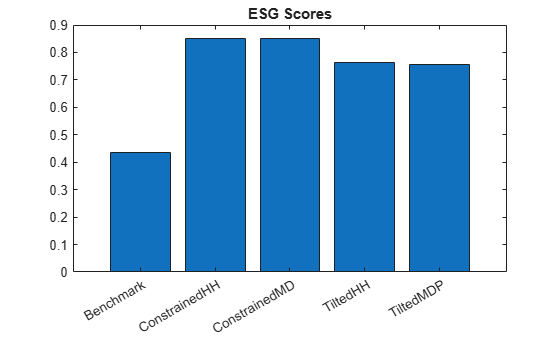As expected, the ESG scores of the penalized strategies are better than the ESG scores of the minimum variance portfolio without constraint and penalty terms. However, the tilted strategies achieve lower ESG scores than the ones that include the target ESG score as a constraint. This comparison shows the flexibility of the tilted strategy. If the ESG target score is not an essential requirement, then you can consider a tilting strategy.

### Backtest Using Strategies

To show the performance through time for the two strategies (Diversification for Fixed ESG Level and Diversification by "Tilting"), use the `backtestEngine` framework. Use `backtestStrategy` to compare the strategies with a prespecified target ESG score with the strategies that use ESG tilting.

```% Store info to pass to ESG constrained strategies Ain = -ESGnumeric'; bin = -ESG0; % Set target ESG score conStruct.p = setInequality(p,-ESGnumeric',-ESG0); conStruct.ret0 = ret0; conStruct.lambdaHH =0.01; conStruct.lambdaMDP =0.05; % Store info to pass to ESG tilting strategies tiltStruct.p = setInequality(p,[],[]); tiltStruct.ret0 = ret0; % HH tilting penalty parameters % Penalty parameter for assets with 'low' ESG score HHlambdaLow =0.1; % Penalty parameter for assets with 'high' ESG score HHlambdaHigh =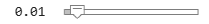0.01; % Combined penalty terms for HH tiltStruct.lambdaHH = (ESG.ESGlabel=='low').*HHlambdaLow + ... (ESG.ESGlabel=='high').*HHlambdaHigh; % MDP tilting penalty parameters % Penalty parameter for assets with 'low' ESG score MDPlambdaLow =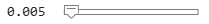0.005; % Penalty parameter for assets with 'high' ESG score MDPlambdaHigh =0.05; % Combined penalty terms for MDP tiltStruct.lambdaMDP = (ESG.ESGlabel=='low').*MDPlambdaLow + ... (ESG.ESGlabel=='high').*MDPlambdaHigh;```

Define the investment strategies that you want to use to make the asset allocation decisions at each investment period. For this example, four investment strategies are defined as input to `backtestStrategy`. The first two strategies require a minimum ESG score and the last two use the ESG tilting method.

```% Define backtesting parameters warmupPeriod = 84; % Warmup period rebalFreq = 42; % Rebalance frequency lookback = [42 126]; % Lookback window transactionCost = 0.001; % Transaction cost for trade % Constrained variance + HH strategy strat1 = backtestStrategy('MixedHH', @(w,P) MixHH(w,P,conStruct), ... 'RebalanceFrequency', rebalFreq, ... 'LookbackWindow', lookback, ... 'TransactionCosts', transactionCost, ... 'InitialWeights', wHHMix); % Constrained variance + MDP strat2 = backtestStrategy('MixedMDP', @(w,P) MixMDP(w,P,conStruct), ... 'RebalanceFrequency', rebalFreq, ... 'LookbackWindow', lookback, ... 'TransactionCosts', transactionCost, ... 'InitialWeights', wMDPMix); % HH tilted strategy strat3 = backtestStrategy('TiltedHH', @(w,P) tiltedHH(w,P,tiltStruct), ... 'RebalanceFrequency', rebalFreq, ... 'LookbackWindow', lookback, ... 'TransactionCosts', transactionCost, ... 'InitialWeights', wTiltHH); % MDP tilted strategy strat4 = backtestStrategy('TiltedMDP', @(w,P) tiltedMDP(w,P,tiltStruct), ... 'RebalanceFrequency', rebalFreq, ... 'LookbackWindow', lookback, ... 'TransactionCosts', transactionCost, ... 'InitialWeights', wTiltMDP); % All strategies strategies = [strat1,strat2,strat3,strat4];```

Run the backtest using `runBacktest` and generate a `summary` for each strategy's performance results.

```% Create the backtesting engine object backtester = backtestEngine(strategies); % Run backtest backtester = runBacktest(backtester,assetPrices,... 'Start',warmupPeriod); % Summary summary(backtester)```
```ans=9×4 table MixedHH MixedMDP TiltedHH TiltedMDP __________ __________ __________ __________ TotalReturn 0.7077 1.1927 0.79527 1.3295 SharpeRatio 0.029317 0.03188 0.031911 0.034608 Volatility 0.011965 0.017845 0.011719 0.016851 AverageTurnover 0.0054528 0.0065605 0.0053991 0.0070898 MaxTurnover 0.80059 0.72624 0.80099 0.76676 AverageReturn 0.00035068 0.00056875 0.00037387 0.00058304 MaxDrawdown 0.23083 0.34334 0.22526 0.34688 AverageBuyCost 0.068778 0.097277 0.070048 0.12019 AverageSellCost 0.068778 0.097277 0.070048 0.12019 ```

To visualize their performance over the entire investment period, plot the daily results of the strategies using `equityCurve`.

```% Plot daily stocks and ESG strategy behavior figure t = tiledlayout(2,1,'Padding','none'); % Equity curves nexttile(t) equityCurve(backtester); % Table and plot of the average ESG score for the mixed and tilted % strategies throughout the investment period nexttile(t) TAvgESG = averageESGTimetable(backtester,ESGnumeric,ESG0);```In the Equity Curve plot, the tilted MDP strategy is the one that performs the best by the end of the investment period, followed by the mixed MDP strategy with the ESG constraint. The performance of the mixed and tilted strategies depend on the choice of the penalty parameters. Both ESG methods, the constrained and the tilted methods, require defining two parameters for each strategy. The ESG constrained method requires you to provide a target ESG score and a penalty parameter for the diversification term. The ESG tilting requires one penalty parameter value for the assets with `'high'` ESG scores and a different one for `'low'` ESG scores. In addition, the ESG tilting requires a third parameter to determine the cutoff point between `'high'` and `'low'` ESG assets. Given the dependency of the penalized strategies on the value of their parameters, the performance of those strategies vary widely. However, this example shows that it is possible to find values of the parameters so that the resulting strategies obtain good returns while improving on the average ESG score.

The ESG Curves plot shows the average ESG evolution computed throughout the investment period for the penalized investment strategies, both for the ESG constrained method and the ESG tilting method. You can see that, unlike the choice of the ESG target for the ESG constraint, the selection of the tilting penalty parameters has an effect on the ESG score of the optimal portfolios that is less explicit. Therefore, the average ESG score varies more with the tilting strategies than with the constrained strategies, as expected.

Although not shown in this example, the traditional minimum variance portfolio strategy, subject to the same expected return and ESG levels, results in higher average turnover and transaction costs than any of the strategies covered in the example. Another advantage of adding a diversification measure to the formulation of the problem is to reduce the average turnover and transaction costs.

### References

 Richard, J. C., and T. Roncalli. Smart Beta: Managing Diversification of Minimum Variance Portfolios. Risk-Based and Factor Investing. Elsevier, pp. 31-63, 2015.

### Local Functions

```function [] = plotESGContours(p,ESGscores,minESG,maxESG,nCont,... nPort) % Plot mean-variance frontier for different ESG levels % Add ESG constraint p.AInequality = -ESGscores'; % Compute mean-variance risks and returns for different ESG levels contourESG = linspace(minESG,maxESG,nCont+1); figure hold on labels = strings(nCont+1,1); for i = 1:nCont p.bInequality = -contourESG(i); % Change target ESG score plotFrontier(p,nPort); labels(i) = sprintf("%6.2f ESG",contourESG(i)*100); end % Plot the original mean-variance frontier p.AInequality = []; p.bInequality = []; plotFrontier(p,nPort); labels(i+1) = "No ESG restriction"; title('Efficient Frontiers') legend(labels,'Location','southeast') hold off end function [ESGTimetable] = averageESGTimetable(backtester,... ESGscores,targetESG) % Create a table of the average ESG score for the mix and tilted % strategies throughout the investment period and plot it % Normalize weights wMixedHH = backtester.Positions.MixedHH{:,2:end}./... sum(backtester.Positions.MixedHH.Variables,2); wMixedMDP = backtester.Positions.MixedMDP{:,2:end}./... sum(backtester.Positions.MixedMDP.Variables,2); wTiltedHH = backtester.Positions.TiltedHH{:,2:end}./... sum(backtester.Positions.TiltedHH.Variables,2); wTiltedMDP = backtester.Positions.TiltedMDP{:,2:end}./... sum(backtester.Positions.TiltedMDP.Variables,2); % Compute ESG scores for the different strategies consHH_ESG = wMixedHH*ESGscores; consMDP_ESG = wMixedMDP*ESGscores; tiltedHH_ESG = wTiltedHH*ESGscores; tiltedMDP_ESG = wTiltedMDP*ESGscores; % Create timetable ESGTimetable = timetable(backtester.Positions.TiltedHH.Time,... consHH_ESG,consMDP_ESG,tiltedHH_ESG,tiltedMDP_ESG); % Plot ESG curves plot(ESGTimetable.Time, ESGTimetable.Variables); hold on plot(ESGTimetable.Time, targetESG*ones(size(ESGTimetable.Time)),... 'k--','LineWidth',1); % Plots target ESG scores title('ESG Curves'); ylabel('Averagde ESG Score'); legend('MixedHH','MixedMDP','TiltedHH','TiltedMDP', 'TargetESG',... 'Location','southwest'); end function new_weights = MixHH(~, assetPrices, struct) % Min variance + max HH diversification strategy % Retrieve portfolio information p = struct.p; ret0 = struct.ret0; % Define returns and covariance matrix assetReturns = tick2ret(assetPrices); p = estimateAssetMoments(p,assetReturns{:,:}); % Objective function: Variance + Herfindahl-Hirschman % diversification term % min x'*Sigma*x + lambda*x'*x objFun = @(x) x'*p.AssetCovar*x + struct.lambdaHH*(x'*x); % Solve problem % Solution that minimizes the variance + HH index new_weights = estimateCustomObjectivePortfolio(p,objFun,... TargetReturn=ret0); end function new_weights = MixMDP(~, assetPrices, struct) % Min variance + MDP diversification strategy % Retrieve portfolio information p = struct.p; ret0 = struct.ret0; % Define returns and covariance matrix assetReturns = tick2ret(assetPrices); p = estimateAssetMoments(p,assetReturns{:,:}); sigma = sqrt(diag(p.AssetCovar)); % Objective function: Variance + MDP diversification term % min x'*Sigma*x - lambda*sigma'*x objFun = @(x) x'*p.AssetCovar*x - struct.lambdaMDP*(sigma'*x); % Solve problem % Solution that minimizes variance + MDP term new_weights = estimateCustomObjectivePortfolio(p,objFun,... TargetReturn=ret0); end function new_weights = tiltedHH(~,assetPrices,struct) % Tilted HH approach % Retrieve portfolio information p = struct.p; ret0 = struct.ret0; lambda = struct.lambdaHH; % Define returns and covariance matrix assetReturns = tick2ret(assetPrices); p = estimateAssetMoments(p,assetReturns{:,:}); % Objective function: Variance + Herfindahl-Hirschman % diversification term % min x'*Sigma*x + lambda*x'*x objFun = @(x) x'*p.AssetCovar*x + lambda'*(x.^2); % Solve problem % Solution that minimizes variance + HH term new_weights = estimateCustomObjectivePortfolio(p,objFun,... TargetReturn=ret0); end function new_weights = tiltedMDP(~,assetPrices,struct) % Tilted MDP approach % Retrieve portfolio information p = struct.p; ret0 = struct.ret0; lambda = struct.lambdaMDP; % Define returns and covariance matrix assetReturns = tick2ret(assetPrices); p = estimateAssetMoments(p,assetReturns{:,:}); sigma = sqrt(diag(p.AssetCovar)); % Objective function: Variance + MDP % min x'*Sigma*x - lambda*sigma'*x objFun = @(x) x'*p.AssetCovar*x - lambda'*(sigma.*x); % Solve problem % Solution that minimizes variance + MDP term new_weights = estimateCustomObjectivePortfolio(p,objFun,... TargetReturn=ret0); end % The API of the rebalance functions (MixHH, MixMDP, tiltedHH, and % tiltedMDP) require a first input with the current weights. They % are redundant for these strategies and can be ignored.```